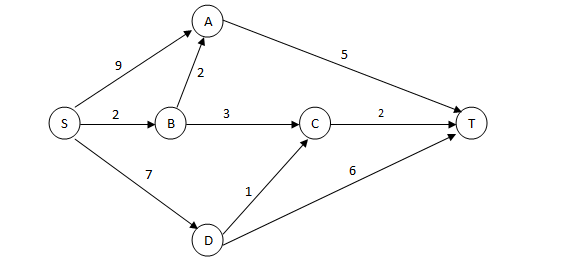### CAT 2006 Question Paper Question 44

Instructions

A significant amount of traffic flows from point S to point T in the one-way street network shown below. Points A, B, C, and D are junctions in the network, and the arrows mark the direction of traffic flow. The fuel cost in rupees for travelling along a street is indicated by the number adjacent to the arrow representing the street. –Motorists traveling from point S to point T would obviously take the route for which the total cost of traveling is the minimum. If two or more routes have the same least travel cost, then motorists are indifferent between them. Hence, the traffic gets evenly distributed among all the least cost routes.

The government can control the flow of traffic only by levying appropriate toll at each junction. For example, if a motorist takes the route S-A-T (using junction A alone), then the total cost of travel would be Rs 14 (i.e., Rs 9 + Rs 5) plus the toll charged at junction A.

Question 44

# If the government wants to ensure that the traffic at S gets evenly distributed along streets from S to A, from S to B, and from S to D, then a feasible set of toll charged (in rupees) at junctions A, B, C, and D respectively to achieve this goal is:

Solution

Total cost = fuel cost + toll
Total cost along SAT : 14+tollA
Total cost along SBAT : 9+tollA+tollB
Total cost along SDT : 13+tollD
Now when option A is considered , total costs come out to be same.
​Hence option A is correct.